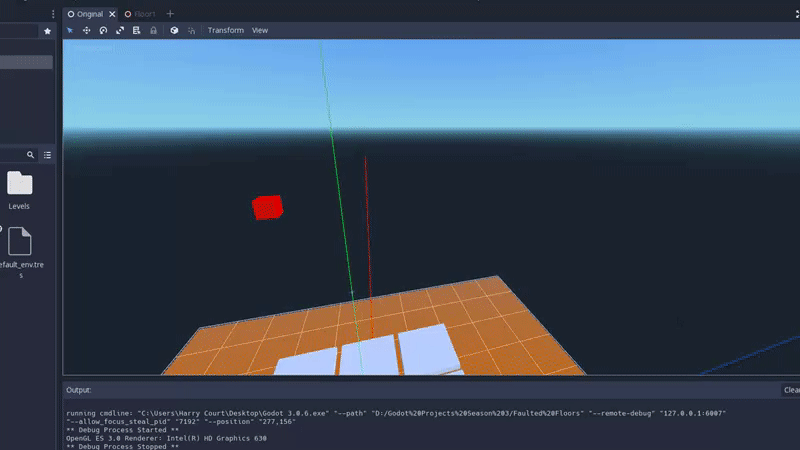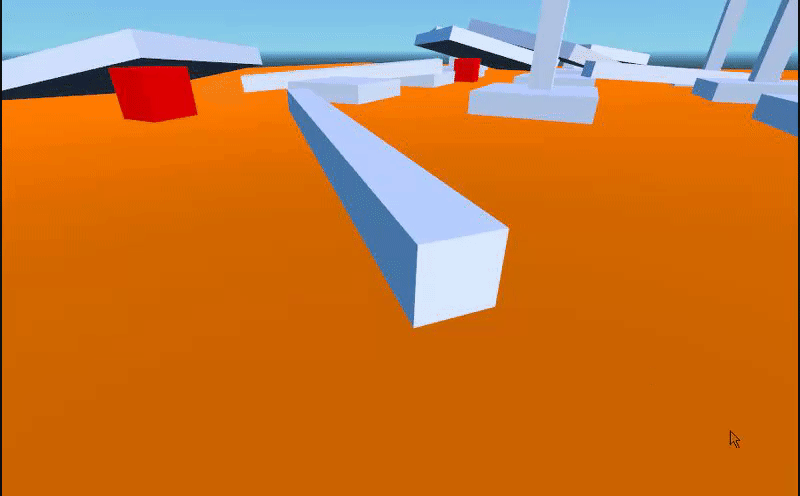Warning, lots of text incoming!

Hello everyone! I'm making a game that involves platforms that collapse when an object hits it, like a boulder. However, I have two issues;

Bug #1:Whenever the player collides with a platform that has been hit by a block or another platform, the player can unbalance it. I have tried removing the mask and layer collision for the platform and pole holding it, but to no avail.

Increasing gravity makes the platforms jitter a bit, and I use "Is Sleeping" to make sure that the player (A KinematicBody) doesn't make it tumble if the platform hasn't collided with a rigid body anything.

Bug #2:I suspect that if bug #1 is fixed, it should fix #2. The problem is similar to the first one, and that the player can push everything.

Here is the player code (Which is a bit old, but works):

``````    extends KinematicBody

# Cameras
var view_sensitivity = 1.5 / 10
var camera_angle = 0
var camera_change = Vector2()

# Vectors
var velocity = Vector3()
var direction = Vector3()

# Moving
var gravity = -9.8 * 3
const MAX_SPEED = 5
const MAX_RUNNING_SPEED = 10
const MAX_CROUCHING_SPEED = 2
const ACCEL = 10
const DEACCEL = 10
var jump_height = 10
var canSprint = true

\$PlayerMesh.hide()

func _physics_process(delta):
walk(delta)

# The chunk of code that makes the player move.
func walk(delta):
var speed

direction = Vector3()

# Walking
if Input.is_action_pressed("move_up"):
direction -= aim.z
if Input.is_action_pressed("move_down"):
direction += aim.z
if Input.is_action_pressed("move_left"):
direction -= aim.x
if Input.is_action_pressed("move_right"):
direction += aim.x

direction.y = 0
direction = direction.normalized()

speed = MAX_SPEED

direction = direction.normalized()

velocity.y += gravity * delta

var temp_velocity = velocity
temp_velocity.y = 0

if Input.is_action_pressed("move_sprint") && canSprint:
speed = MAX_RUNNING_SPEED

var target = direction * speed

var acceleration
if direction.dot(temp_velocity) > 0:
acceleration = ACCEL
else:
acceleration = DEACCEL

temp_velocity = temp_velocity.linear_interpolate(target, acceleration * delta)

velocity.x = temp_velocity.x
velocity.z = temp_velocity.z

velocity = move_and_slide(velocity, Vector3(0, 1, 0))

if Input.is_action_pressed("move_jump") && is_on_floor():
velocity.y = jump_height

func death():
pass

func _input(event):

if event is InputEventMouseMotion: